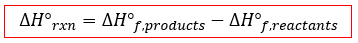# Problem: The standard enthalpy of combustion of naphthalene is -5157 kJ/mol. Calculate its standard enthalpy of formation.The reaction for the combustion of naphthalene is:C10H8 (s) + 12 O2 (g) → 10CO2 (g) + 4 H2O (l)

###### FREE Expert Solution

We’re asked to calculate the standard enthalpy of formation (Δf) for naphthalene (C10H8) based on the standard enthalpy of combustion (Δrxn) and the chemical equation.

Recall that Δrxn can be calculated from the reactants and products involved:We’re given the Δrxn for the combustion of 1 mole of C10H8. The chemical equation for that reaction is:

C10H8(s) + 12 O2(g)  10CO2(g) + 4 H2O(l)

First, we make sure the equation is balanced by checking that the number of elements on both sides is equal.

Balance C: we have 10 C on the reactant side and 10 C on the products side.

Balance H: we have 8 H on the reactant side and 4(2) = 8 H on the products side.

Balance O: we have 12(2) = 24 O on the reactant side and 10(2) + 4(1) = 24 O on the products side.###### Problem Details

The standard enthalpy of combustion of naphthalene is -5157 kJ/mol. Calculate its standard enthalpy of formation.

The reaction for the combustion of naphthalene is:

C10H8 (s) + 12 O2 (g) → 10CO2 (g) + 4 H2O (l)